﻿ 用于高分辨率宽测绘带SAR系统的SAR/GMTI处理方法研究
«上一篇文章快速检索 高级检索

 雷达学报2015, Vol. 4Issue (4): 375–385  DOI: 10.12000/JR150960

### 引用本文 [复制中英文]

[复制中文]
Xing Meng-dao, Sun Guang-cai, Li Xue-shi, et al. Study on SAR/GMTI Processing for High-resolution Wide-swath SAR System[J]. Journal of Radars, 2015, 4(4): 375–385. DOI: 10.12000/JR15096.
[复制英文]

### 文章历史(西安电子科技大学雷达信号处理国家重点实验室 西安 710071)

Study on SAR/GMTI Processing for High-resolution Wide-swath SAR System
(National Laboratory of Radar Signal Processing, Xidian University, Xi'an 710071, China)
Foundation Items: The National Natural Science Foundation of China (61222108, 61301292, 61101245); Fundamental Research Funds for the Central Universities (K5051302058, K5051302046)
Abstract: In recent years, there have been many studies on Synthetic Aperture Radar (SAR), and High- Resolution and Wide-Swath (HRWS) imaging has attracted increasing attention. High-resolution images of a stationary scene enabling the detection of moving targets can be provided by an SAR system combined with Ground Moving Target Indication (GMTI), which is widely used in many civilian applications. A multichannel in azimuth HRWS SAR system has been presented to effectively deal with conflicts between high resolution and low Pulse Repetition Frequency (PRF), which are encountered during HRWS SAR imaging. Because the spatial degrees of freedom in the azimuth can be employed to suppress a clutter, the multichannel configuration offers significant potential for GMTI capability. In this study, a new clutter suppression and moving target imaging method is proposed, which can be operated in an HRWS SAR system without the need for a separate high PRF operation mode.
Key words: Synthetic Aperture Radar (SAR)     High-Resolution Wide-Swath (HRWS)     Moving targets detection     Moving targets imaging     Simultaneous
1 引言

2 HRWS MC-SAR系统的几何关系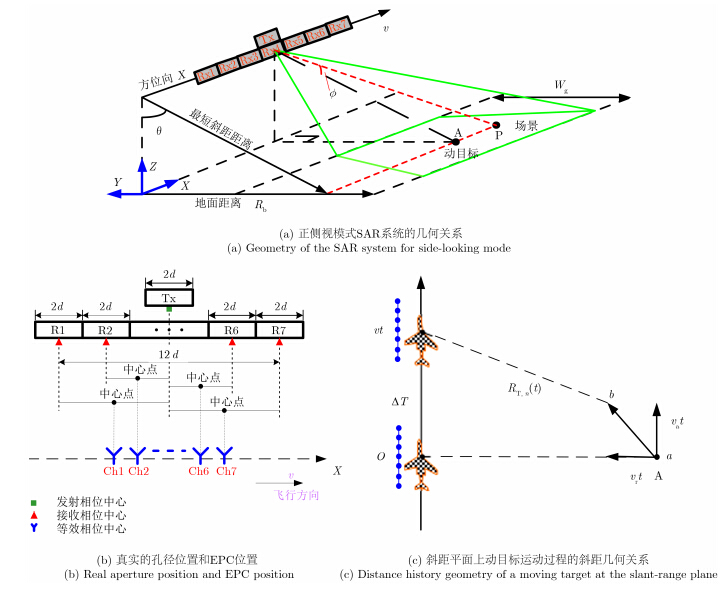图 1 HRWS MC-SAR系统的几何关系 Fig.1 Geometry of an MC-HRWS SAR system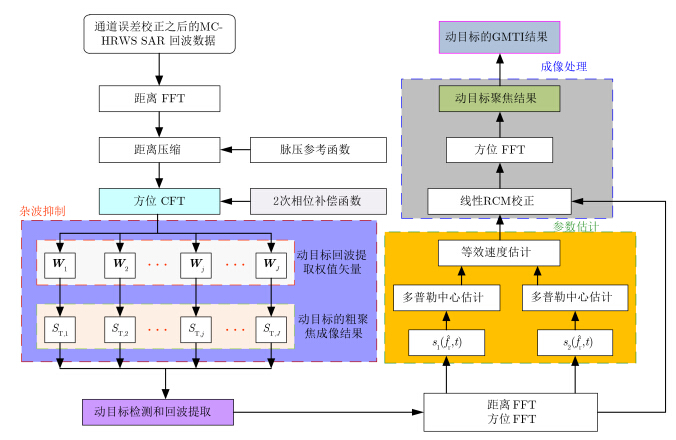图 2 本文所提HRWS MC-SAR中杂波抑制和动目标成像算法流程图 Fig.2 Flowchart of the proposed clutter suppression and moving target imaging algorithm for an MC-HRWS SAR system
3 动目标和杂波的粗聚焦图像及复响应

CFT作为FT的2阶推广，比原始的FT具有更好处理线性调频信号的能力。信号s(t)的CFT可以表示为
 \begin{align} S\left( {{f \! _{\rm a}}} \right) = & \int {s\left( t \right)\exp \left\{ { - {\rm{j}}\left( {{{\varphi _{_0}}} + 2{π} {f_1}t + {π} {k_{\rm a}}{t^2}} \right)} \right\}} \\ & \cdot \exp \left( { - {\rm{j}}2{π} {f_{\mathop{\rm a}\nolimits} }t} \right){\rm{d}}t \end{align} (1)

 \begin{align} s\left( t \right) = & \frac{1}{{2{π} }}\int {S\left( {{f \! _{\rm a}}} \right)\exp \left\{ {{\rm{j}}\left( {{\varphi _0} + 2{π} {f_1}t + {π} {k_{\rm a}}{t^2}} \right)} \right\}} \\ & \cdot \exp \left( {{\rm{j}}2{π} {f \! _{\rm a}}t} \right){\rm{d}}{f \! _{\rm a}} \end{align} (2)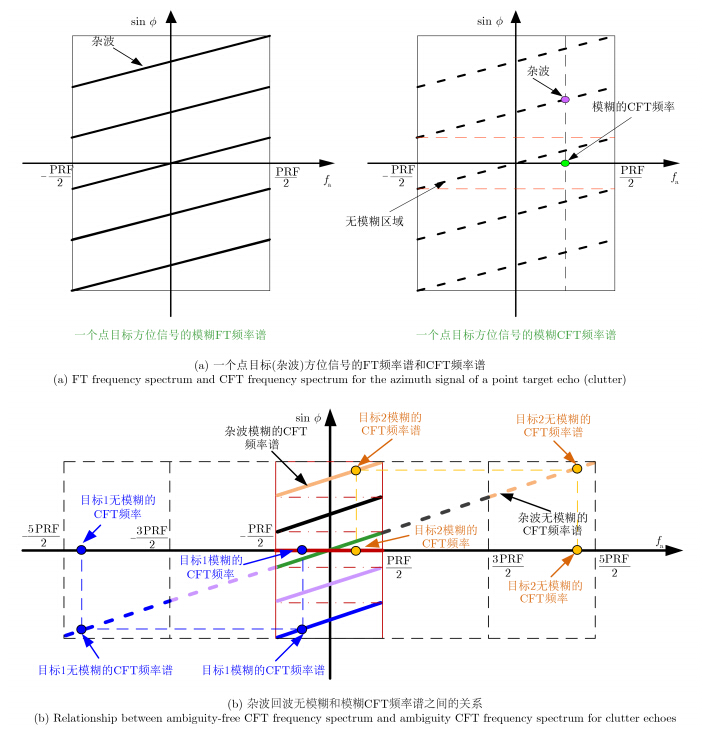图 3 杂波回波方位信号的CFT Fig.3 CFT for the azimuth signal of clutter echoes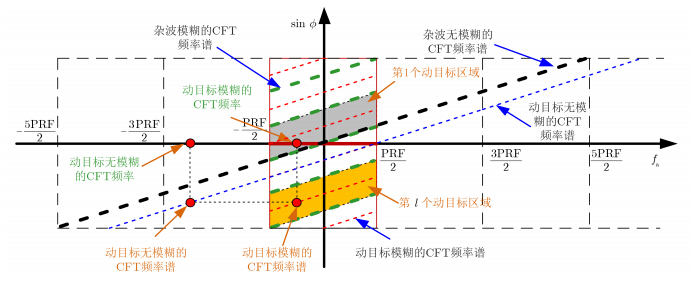图 4 杂波回波方位信号的CFT Fig.4 CFT for the azimuth signal of clutter echoes
4 杂波抑制和动目标检测

 $\begin{array}{*{20}{l}} {\mathop {\min }\limits_{{W_{\rm{l}}}} W_{\rm{l}}^{\rm{H}}\left( {{f_{\rm{a}}}} \right){R_{\rm{X}}}\left( {{f_{\rm{a}}}} \right){W_{\rm{l}}}\left( {{f_{\rm{a}}}} \right)}\\ {{\rm{s}}.{\rm{t}}\;W_{\rm{l}}^{\rm{H}}\left( {{f_{\rm{a}}}} \right)C = Q} \end{array}\}$ (3)

 ${R_X}({f_a}) = E[({S_c}({f_a}) + {S_T}({f_a})){({S_c}({f_a}) + {S_T}({f_a}))^H}]$ (4)

 $C = [{a_{T,l}}({f_a}),{a_{c, - L}}({f_a}), \cdots ,{a_{c,l}}({f_a}), \cdots ,{a_{c,L}}({f_a})]$ (5)

 ${W}_{\rm l}^{\rm opt}\left( {{f \! _{\rm a}}} \right) = {R}_{\rm X}^{ - 1}{C}{\left( {{{C}^{\rm{H}}}{R}_{\rm X}^{ - 1}{C}} \right)^{ - 1}}{Q}$ (6)

 ${{\hat S}_{{\rm{T}},{\rm{l}}}}\left( {{f_{\rm{a}}}} \right) = {\left[ {W_{\rm{l}}^{{\rm{opt}}}\left( {{f_{\rm{a}}}} \right)} \right]^{\rm{H}}} \cdot \left( {{S_{\rm{c}}}\left( {{f_{\rm{a}}}} \right) + {S_{\rm{T}}}\left( {{f_{\rm{a}}}} \right)} \right)$ (7)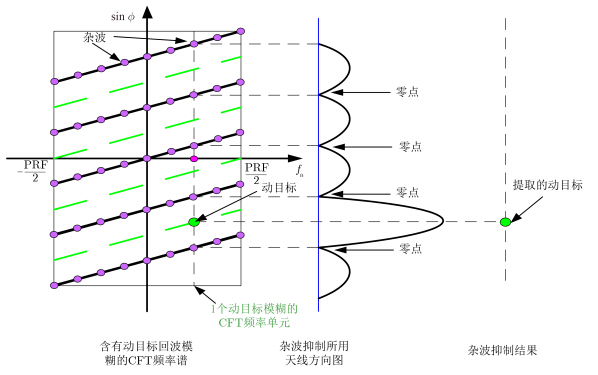图 5 本文所提出的杂波抑制算法处理过程 Fig.5 Clutter suppression processing of the proposed approach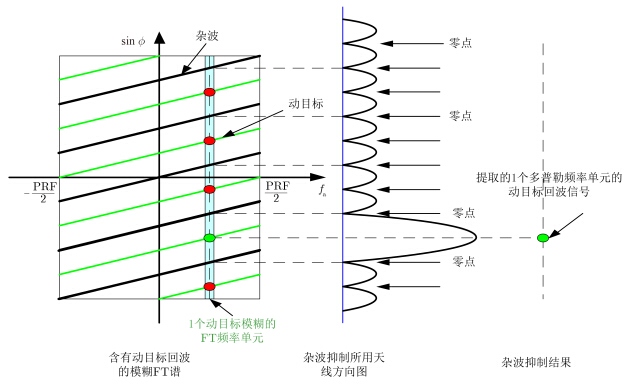图 6 ISTAP方式的杂波抑制处理和动目标信号提取 Fig.6 Clutter suppression processing and moving target signal extraction of the ISTAP approach
5 动目标成像和斜距速度估计

 \begin{align} {s_{\rm T}}\left( {{f_{\rm r}},t} \right) \! = & {\sigma _{\rm T}}{G_{\rm L}}{W_{\rm r}}\left( {{f_{\rm r}}} \right){w_{\rm az}}\left( {t - {t_{\rm T}}} \right)\\ & \! \cdot \exp \! \left\{ { - {\rm{j}}\frac{{4{π} }}{{\rm{c}}}\left( {{f_{\rm c}} + {f_{\rm r}}} \right){v_{\rm r\_equ}}\left( {t - {t_0}} \right)} \right\}\\ & \! \cdot \exp \! \left\{ { \! - {\rm{j}}\frac{{4{π}}}{{\rm{c}}}\left( {{f_{\rm{c}}} \! + \! {f_{\rm{r}}}} \right) \! \left( {{\rm{ }}{R_{{\rm{equ}}\_{\rm{T}}}} \! + \! {v_{\rm{r}}}\left( {{t_0} \! - \! {t_{\rm{T}}}} \right)} \right)} \! \right\} \end{align} (8)

 ${\hat f_{\rm dc\_1}} = \frac{{2{v_{\rm r\_equ}}}}{{\rm{c}}}\left( {{f_{\rm c}} - \frac{B}{4}} \right) - {M_{\rm amb}} \cdot {\mathop{\rm PRF}\nolimits}$ (9)
 ${\hat f_{{\rm{dc\_2}}}} = \frac{{2{v_{\rm r\_equ}}}}{{\rm{c}}}\left( {{f_{\rm c}} + \frac{B}{4}} \right) - {M_{\rm amb}} \cdot {\mathop{\rm PRF}\nolimits}$ (10)

 ${\hat v_{\rm r\_equ}} = \frac{{\left( {{{\hat f}_{\rm dc\_2}} - {{\hat f}_{{\mathop{\rm dc}\nolimits} \_1}}} \right) \cdot {\rm{c}}}}{B}$ (11)

 ${\hat v_{\rm r}} = \frac{{\left( {{{\hat f}_{\rm dc\_2}} - {{\hat f}_{{\mathop{\rm dc}\nolimits} \_1}}} \right) \cdot {\rm{c}}}}{B} - \frac{{{v^2}}}{{{R_0}}}\left( {{t_0} - {t_{\rm T}}} \right)$ (12)

 ${H_2}\left( {{f_{\rm r}},t} \right) = \exp \left\{ {{\rm{j}}\frac{{4{π} }}{{\rm{c}}}{f_{\rm r}} \cdot {{\hat v}_{\rm r\_equ}}\left( {t - {t_0}} \right)} \right\}$ (13)

 \begin{align} {s_{\rm T}}\left( {\hat t,{f \! _{\rm a}}} \right) = & {\sigma _{\rm T}}{G_{\rm L}}{G_{\rm r}}{G_{\rm az}}\\ & \cdot {\mathop{\rm sinc}\nolimits} \left( \! {B\left( \! {\hat t - \frac{{2\left( {{R_{\rm equ\_T}} \!+\! {v_{\rm r}}\left( {{t_0} \!-\! {t_{\rm T}}} \right)} \right)}}{{\rm{c}}}} \! \right)} \right)\\ & \cdot {\mathop{\rm sinc}\nolimits} \left( {{T_{\rm a}}\left( {{f \! _{\rm a}} - \frac{{2 \cdot {f_{\rm c}} \cdot {v_{\rm r\_equ}}}}{{\rm{c}}}} \right)} \right)\\ & \cdot \exp \left( { - {\rm{j}}2{π} \left( {{f \! _{\rm a}}{t_{\rm T}} - \frac{{2{f_{\rm c}}{t_0}}}{{\rm{c}}}} \right)} \right) \end{align} (14)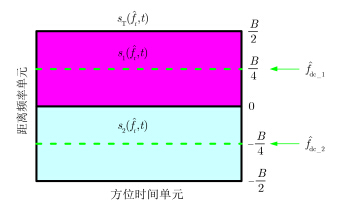图 7 利用提取的动目标回波构造的两组信号 Fig.7 Construction of two groups signals for the extracted moving target echo
6 实测的动目标HRWS MC-SAR数据表 1 实测数据的系统参数 Tab.1 System parameters for the real data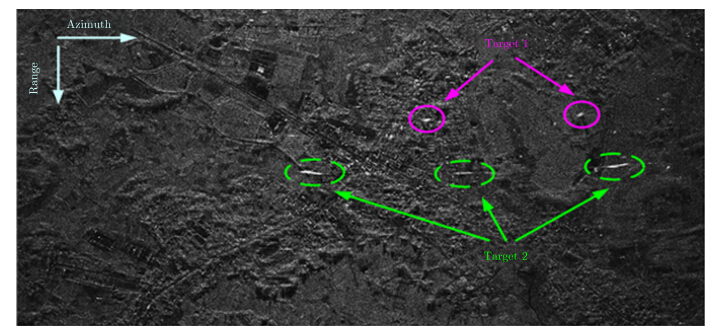图 8 利用参考文献[12, 13, 14]提出的方位多普勒信号重构算法进行重构之后场景回波的成像结果 Fig.8 Imaging result for the scene echoes using the azimuth Doppler signal reconstruction approach proposed in Refs. [12, 13, 14]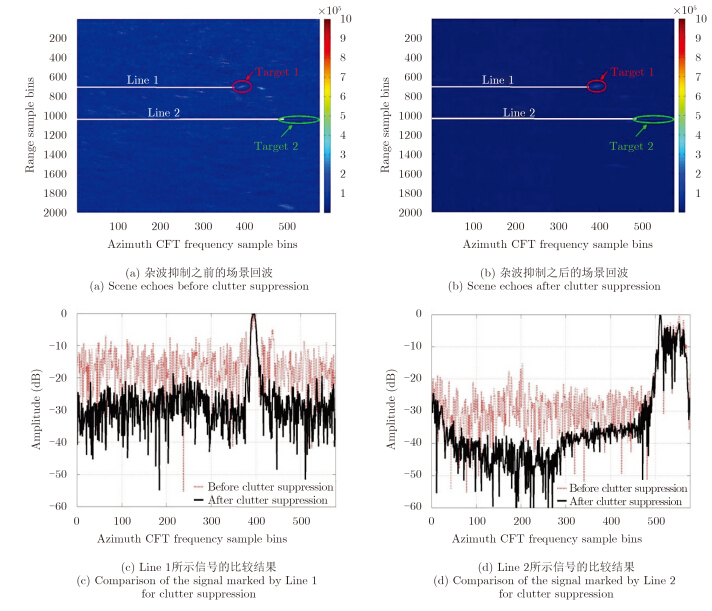图 9 RC-CFT域杂波抑制过程 Fig.9 Clutter suppression process in the RC-CFT domain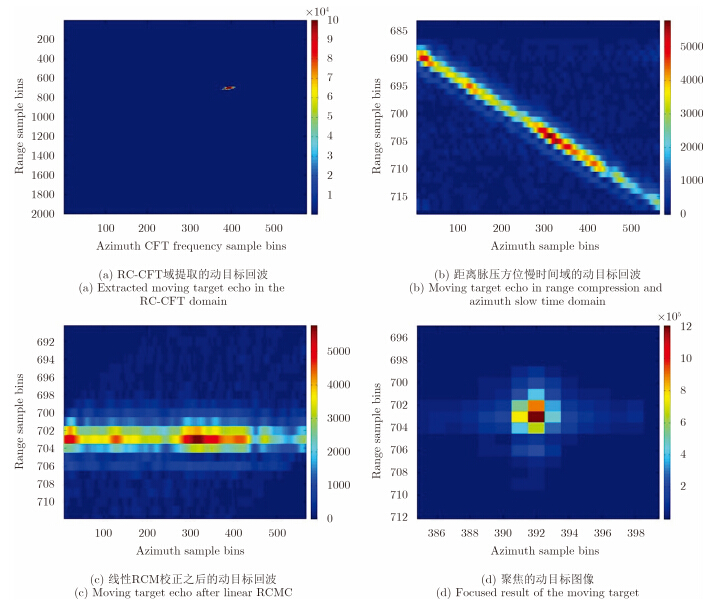图 10 第1个动目标的成像过程 Fig.10 Imaging process of the first moving target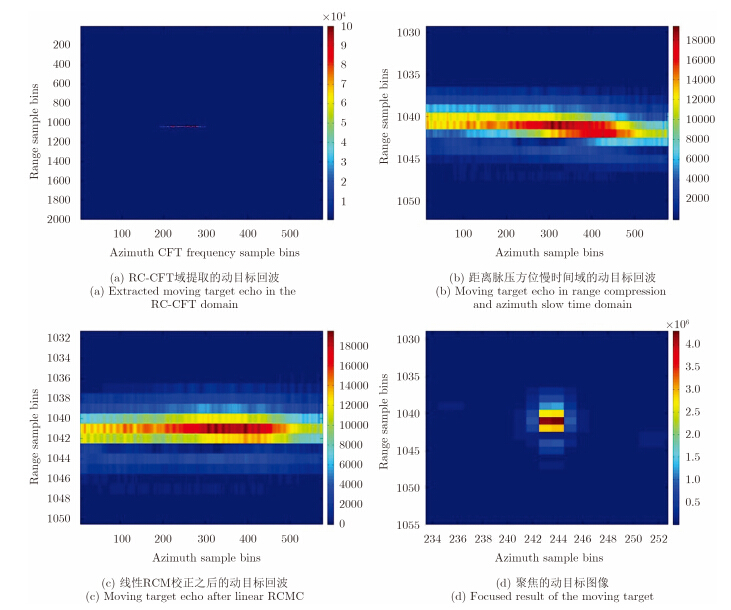图 11 第2个动目标的成像过程 Fig.11 Imaging process of the sceond moving target
7 结束语

  Gierull C H and Sikaneta I C. Raw data based two-aperture SAR ground moving target indication[C]. Proceedings 2003 IEEE IGARSS'03, Toulouse, France, 2003, 2: 1032-1034.(1)  Wang H S C. Mainlobe clutter cancellation by DPCA for space-based Radars[C]. Aerospace Applications Conference, Creasted Butte, CO, USA, 1991: 124-128.(1)  Shen Chiu. Application of fractional Fourier transform to moving target indication via along-track interferometry[J]. EURASIP Journal on Applied Signal Processing, 2005(20): 3293-3303.(1)  Breit H, Eineder M, Holzner J, et al.. Traffic monitoring using SRTM along-track interferometry[C]. Proceedings 2003 IEEE IGARSS'03, 2003, 2: 1187-1189.(1)  Suchandt S, Runge H, Breit H, et al. Automatic extraction of traffic flows using TerraSAR-X along-track interferometry[J]. IEEE Transactions on Geoscience and Remote Sensing, 2010, 48(2): 807–819.(1)  Klemm R. Introduction to space-time adaptive processing[J]. Electronics & Communication Engineering Journal, 1999, 11(1): 5-12.(1)  Zhang S, Xing M, Xia X, et al.. A novel moving target imaging algorithm for multi-channel in azimuth HRWS SAR based on local maximum-likelihood minimum entropy in range frequency[J]. IEEE Transactions on Geoscience and Remote Sensing, 2014, 52(11): 5333-5347.(1)  Xia X G. Discrete chirp-Fourier transform and its application to chirp rate[J]. IEEE Transactions on Signal Processing, 2000, 48(11): 3122-3133.(2)  Madsen S N. Estimating the Doppler centroid of SAR data[J]. IEEE Transactions on Aerospace and Electronic Systems, 1989, 25(2): 134-140.(2)  Cumming I G, Kavanagh P F, Ito M R, et al.. Resolving the Doppler ambiguity for spaceborne synthetic aperture radar[C]. Proceedings 2003 IEEE IGARSS'03, Zurich, Switzerland, 1986: 1639-1643.(2)  Zhang S, Xing M, Xia X, et al.. Multi-channel HRWS SAR imaging based on range-variant channel-calibration and multi-Doppler-direction restriction ambiguity suppression[J]. IEEE Transactions on Geoscience Remote Sensing, 2014, 52(9): 4306-4326.(3)  Delphine C and Ishuwa S. “Optimum GMTI processing for space-based SAR/GMTI systems-Theoretical derivation”[C]. 2010 8th European Conference on Synthetic Aperture Radar, Aachen, Germany, 2010: 390-393.(3)  Cerutti-Maori D and Sikaneta I. Optimum GMTI processing for spacebased SAR/GMTI systems-Simulation results[C]. 2010 8th European Conference on Synthetic Aperture Radar, Aachen, Aachen, Germany, 2010: 653-656.(3)  Cerutti-Maori D, Gierull C H, and Ender J H G. Optimum SAR/GMTI processing and its application to the radar satellite RADARSAT-2 for traffic monitoring[J]. IEEE Transactions on Geoscience and Remote Sensing, 2012, 50(10): 3868-3881.(3)